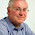# Artificial Intelligence Questions and Answers Set 5

## Artificial Intelligence

### Questions 41 to 50

41.
Which of the following is true related to ‘Satisfiable’ property?
 (a) A statement is satisfiable if there is some interpretation for which it is false (b) A statement is satisfiable if there is some interpretation for which it is true (c) A statement is satisfiable if there is no interpretation for which it is true (d) A statement is satisfiable if there is no interpretation for which it is false (e) None of the above.
42.
Two literals are complementary if
 (a) They are equal (b) They are identical and of equal sign (c) They are identical but of opposite sign (d) They are unequal but of equal sign (e) They are unequal but of opposite sign.
43.
Consider a good system for the representation of knowledge in a particular domain. What property should it possess?
 (a) Representational Adequacy (b) Inferential Adequacy (c) Inferential Efficiency (d) Acquisitional Efficiency (e) All the above.
44.
What is Transposition rule?
 (a) From P → Q, infer ~Q → P (b) From P → Q, infer Q → ~P (c) From P → Q, infer Q → P (d) From P → Q, infer ~Q → ~P (e) None of the above.
45.
Third component of a planning system is to
 (a) Detect when a solution has been found (b) Detect when solution will be found (c) Detect whether solution exists or not (d) Detect whether multiple solutions exist (e) Detect a solutionless system.
46.
Which of the following is true in Statistical reasoning?
 (a) The representation is extended to allow some kind of numeric measure of certainty to be associated with each statement (b) The representation is extended to allow ‘TRUE or FALSE’ to be associated with each  statement (c) The representation is extended to allow some kind of numeric measure of certainty to be associated common to all statements (d) The representation is extended to allow ‘TRUE or FALSE’ to be associated common to all statements (e) None of the above.
47.
In default logic, we allow inference rules of the form
 (a) (A : B) / C (b) A / (B : C) (c) A / B (d) A / B : C (e) (A: B) :C.
48.
In Baye’s theorem, what is the meant by P(Hi|E)?
 (a) The probability that hypotheses Hi is true given evidence E (b) The probability that hypotheses Hi is false given evidence E (c) The probability that hypotheses Hi is true given false evidence E (d) The probability that hypotheses Hi is false given false evidence E (e) The probability that hypotheses Hi is true given unexpected evidence E.
49.
Default reasoning is another type of
 (a) Monotonic reasoning (b) Analogical reasoning (c) Bitonic reasoning (d) Non-monotonic reasoning (e) Closed world assumption.
50.
Generality is the measure of
 (a) Ease with which the method can be adapted to different domains of application (b) The average time required to construct the target knowledge structures from some specified initial structures (c) A learning system to function with unreliable feedback and with a variety of training examples (d) The overall power of the system (e) Subdividing the system.

#### Answers

 41 Answer : (b) Reason  :       ‘Satisfiable’ property is a  statement is satisfiable if there is some interpretation for which it is true. 42 Answer : (c) Reason  :       Two literals are complementary if They are identical but of opposite sign. 43 Answer : (e) Reason  :       Consider a good system for the representation of knowledge in a particular domain. The properties should be Representational Adequacy,Inferential Adequacy,Inferential Efficiency and Acquisitional Efficiency 44 Answer : (d) Reason  :       Transposition rule- From P → Q, infer ~Q → ~P 45 Answer : (a) Reason  :       Third component of a planning system is to detect when a solution has been found. 46 Answer : (a) Reason  :       Statistical reasoning is the representation is extended to allow some kind of numeric measure of certainty to be associated with each statement. 47 Answer : (a) Reason  :       In default logic, we allow inference rules of the form:(A : B) / C 48 Answer : (a) Reason  :       In Baye’s theorem,  P(Hi|E)  is the probability that hypotheses Hi is true given evidence E. 49 Answer : (d) Reason  :       Default reasoning is another type of non-monotonic reasoning 50 Answer : (a) Reason  :       Generality is the measure of ease with which the method can be adapted to different domains of application.

<< Prev  1 ...  2 ...  3 ...  4 ...  5 ...  6 ...  Next  >>

#### 5 comments :

1.The company run by Tim Cook is added to the long list of technology companies that are interested in the field of artificial intelligence. In the list are and Facebook, Google and Microsoft.speech recognition program

2.Afterwards, the creator of the humanoid robot quickly replies with, “Nooo, I take it back, don’t destroy humans!” computer intelligence

3.Good question .useful at the time of interview.Keep updating Artificial Intelligence Online Training

4.Much obliged for Sharing a helpful substance about Artificial Intelligence,Please visit our site At colan infotech we shared a few blogs about AI.
Augmented reality app development company
Best augmented reality companies
Augmented reality developers
Augmented reality development companies
best augmented reality companies
Augmented reality app development company

5.1.Thereispurefree-marketeconomyintheworld.true or false